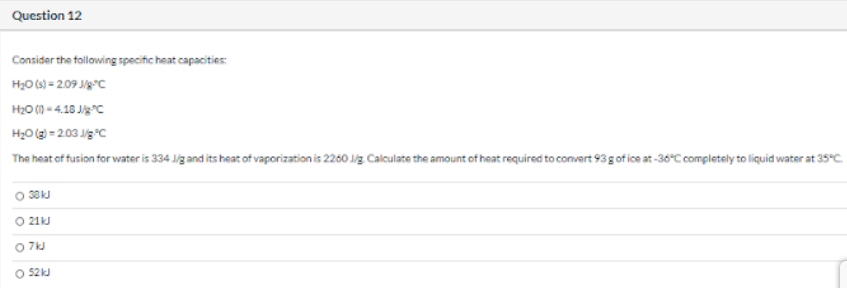# Consider the following specific heat capacities: H2O(s) = 2.09 J/g.°C H2O(l) = 4.18 J/g.°C H2O(g) = 2.03 J/g.°C The heat of fusion for water is 334 J/g and its heat of vaporization is 2260 J/g. Calculate the amount of heat required to convert 93 g of ice at -36°C completely to liquid water at 35°C. a) 38 kJ b) 21 kJ c) 7 kJ d) 52 kJ